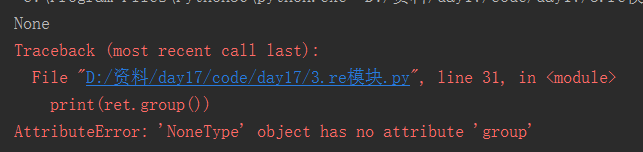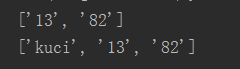# re模块

2019-12-06 10:56

## 爬虫——正则表达式re模块，爬虫正则表达式re

• 正则表达式，又称规则表达式，通常被用来检索、替换那些符合某个规则的文本。
• 正则表达式是对字符串操作的一种逻辑公式，就是用事先定义好的一些特定字符及这些特定字符的组合，组成一个“规则字符串”，这个“规则字符串”用来表达对字符串的一种过滤逻辑。

• 给定的字符串是否符合正则表达式的过滤逻辑（“匹配”）
• 通过正则表达式，从文本字符串中获取我们想要的特定部分（“过滤”）Python的re模块

re模块的一般使用步骤如下：

compile函数

compile函数用于编译正则表达式，生成一个Pattern对象，它的一般使用形式如下：

``````#!/usr/bin/python3
# -*- conding:utf-8 -*-
__author__ = 'mayi'

import re

# 将正则表达式编译成Pattern对象
pattern = re.compile('d ')
``````

Pattern对象的一些常用方法主要有：

• match()方法：从起始位置开始查找，一次匹配
• search()方法：从任何位置开始查找，一次匹配
• findall()方法：全部匹配，返回列表
• finditer()方法：全部匹配，返回迭代器
• split()方法：分割字符串，返回列表
• sub()方法：替换

match()方法

match()方法用于查找字符串的头部（也可以指定起始位置），它是一次匹配，只要找到了一个匹配的结果就返回，而不是查找所有匹配的结果。它的一般使用形式如下：

match(string[, pos[, endpos]])

``````>>> import re
>>> pattern = re.compile(r'd ')  # 用于匹配至少一个数字

>>> m = pattern.match('one12twothree34four')  # 查找头部，没有匹配
>>> print m
None

>>> m = pattern.match('one12twothree34four', 2, 10) # 从'e'的位置开始匹配，没有匹配
>>> print(m)
None

>>> m = pattern.match('one12twothree34four', 3, 10) # 从'1'的位置开始匹配，正好匹配
>>> print(m)                                        # 返回一个 Match 对象
<_sre.SRE_Match object; span=(3, 5), match='12'>

>>> print(m.group(0))   # 可省略 0
12
>>> print(m.start(0))   # 可省略 0
3
>>> print(m.end(0))     # 可省略 0
5
>>> print(m.span(0))    # 可省略 0
(3, 5)
``````

• group([group1,...])方法用于获得一个或多个分组匹配的字符串，当要获得整个匹配的子串时，可直接使用group()或group(0)
• start([group])方法用于获取分组匹配的子串在整个字符串中的起始位置（子串第一个字符的索引），参数默认值为0
• end([group])方法用于获取分组匹配的子串在整个字符串中的结束位置（子串最后一个字符的索引 1），参数默认值为0
• span([group])方法返回(start(group), end(group))

``````>>> import re
>>> pattern = re.compile(r'([a-z] ) ([a-z] )', re.I)  # re.I 表示忽略大小写
>>> m = pattern.match('Hello World Wide Web')

>>> print(m)     # 匹配成功，返回一个 Match 对象
<_sre.SRE_Match object; span=(0, 11), match='Hello World'>

>>> print(m.group(0))  # 返回匹配成功的整个子串
Hello World

>>> print(m.span(0))   # 返回匹配成功的整个子串的索引
(0, 11)

>>> print(m.group(1))  # 返回第一个分组匹配成功的子串
Hello

>>> print(m.span(1))   # 返回第一个分组匹配成功的子串的索引
(0, 5)

>>> print(m.group(2))  # 返回第二个分组匹配成功的子串
World

>>> print(m.span(2))   # 返回第二个分组匹配成功的子串
(6, 11)

>>> print(m.groups())  # 等价于 (m.group(1), m.group(2), ...)
('Hello', 'World')

>>> print(m.group(3))   # 不存在第三个分组
Traceback (most recent call last):
File "<stdin>", line 1, in <module>
IndexError: no such group
``````

search()方法

search()方法用于查找字符串的任何位置，它也是一次匹配，只要找到了一个匹配的结果就返回，而不是查找所有匹配的结果，它的一般使用形式如下：

search(string[, pos[, endpos]])

``````>>> import re
>>> pattern = re.compile('d ')
>>> m = pattern.search('one12twothree34four')
>>> print(m)
<_sre.SRE_Match object; span=(3, 5), match='12'>
>>> print(m.group())
12
>>> m = pattern.search('one12twothree34four', 10, 30)
>>> print(m)
<_sre.SRE_Match object; span=(13, 15), match='34'>
>>> print(m.group())
34
>>> print(m.span())
(13, 15)
``````

``````#!/usr/bin/python3
# -*- conding:utf-8 -*-
__author__ = 'mayi'

import re

# 将正则表达式编译成Pattern对象
pattern = re.compile(r'd ')

# 使用search() 查找匹配的子串，不存在匹配的子串时，返回None
m = pattern.search('hello 123 456 789') # 若这里使用match()，返回None

if m:
print("matching string:", m.group())
print("position:", m.span())
``````

``````matching string: 123
position: (6, 9)
``````

findall()方法

findall()方法的使用形式如下：

findall(string[, pos[, endpos]])

findall()以列表形式返回全部能匹配的子串，如果没有匹配成功，则返回一个空列表。

``````>>> import re
>>> pattern = re.compile(r'd ')  # 匹配数字
>>> res1 = pattern.findall('hello 123 456 789')
>>> res2 = pattern.findall('one1two2three3four4', 0, 16)
>>> print(res1)
['123', '456', '789']
>>> print(res2)
['1', '2', '3']
``````

``````>>> import re
>>> pattern = re.compile(r'd .d ')  # 匹配小数
>>> res = pattern.findall("3.1415926, 'big', 110, 95.5")
>>> print(res)
['3.1415926', '95.5']
``````

finditer()方法

finditer()方法的行为跟findall()的行为类似，也是搜索整个字符串，获得所有匹配的结果。但它返回一个顺序访问每一个匹配结果（Match对象）的迭代器。

``````#!/usr/bin/python3
# -*- conding:utf-8 -*-
__author__ = 'mayi'

import re

pattern = re.compile(r'd ')

res_iter1 = pattern.finditer("hello 123 456 789")
res_iter2 = pattern.finditer("one1two2three3four4", 0, 16)

print(res_iter1)
print(res_iter2)

print("res_iter1......")
for m1 in res_iter1:
print("matching string:{}, position:{}".format(m1.group(), m1.span()))

print("res_iter2......")
for m2 in res_iter2:
print("matching string:{}, position:{}".format(m2.group(), m2.span()))
``````

``````<callable_iterator object at 0x00ADF7F0>
res_iter1......
matching string:123, position:(6, 9)
matching string:456, position:(10, 13)
matching string:789, position:(14, 17)
res_iter2......
matching string:1, position:(3, 4)
matching string:2, position:(7, 8)
matching string:3, position:(13, 14)
``````

split()方法

spilt()方法按照能够匹配的子串将字符串分割后返回列表，它的使用形式如下：

split(string[, maxsplit])

``````>>> import re
>>> pattern = re.compile(r'[s,;] ')
>>> print(pattern.split('a,b;; c  d'))
['a', 'b', 'c', 'd']
``````

sub()方法

sub()方法用于替换。它的使用形式如下：

sub(repl, string[, count])

• 如果repl是字符串，则会使用repl去替换字符串每一个匹配的子串，并返回替换后的字符串，另外，repl还可以使用id的形式来引用分组，但不能使用编号0
• 如果repl是函数，这个方法应当只接受一个参数（Match对象），并返回一个字符串用于替换（返回的字符串中不能再引用分组）
• count用于指定最多替换次数，默认全部替换

``````#!/usr/bin/python3
# -*- conding:utf-8 -*-
__author__ = 'mayi'

import re

pattern = re.compile(r'(w ) (w )') # w: [A-Za-z0-9]

string = 'hello 123, hello 456'

print(pattern.sub('hello world', string))
# 我是分割线
print("*" * 30)

print(pattern.sub(r'2 1', string))

# 我是分割线
print("*" * 30)

def func(m):
return 'hi '   m.group(2)

print(pattern.sub(func, string))

# 我是分割线
print("*" * 30)

# 最多替换一次
print(pattern.sub(func, string, 1))
``````

``````hello world, hello world
******************************
123 hello, 456 hello
******************************
hi 123, hi 456
******************************
hi 123, hello 456
``````

``````#!/usr/bin/python3
# -*- conding:utf-8 -*-
__author__ = 'mayi'

import re

string = "你好，世界。hello world!"

pattern = re.compile(r"[u4e00-u9fa5] ")

res = pattern.findall(string)

print(res)
``````

``````['你好', '世界']
``````

# 三丶match

search:匹配到就返回一个变量,通过group取匹配到的第一个值,匹配不到就返回None,group会报错

### 对于正则表达式来说,有些时候我们需要进行分组,来整体约束y一组字符出现的次数

``````import re
s = '<a>wahaha</a>'  # 标签语言 html 网页
ret = re.search('<(w )>(w )</(w )>',s)
print(ret.group())  # 所有的结果
print(ret.group(1)) # 数字参数代表的是取对应分组中的内容  a
print(ret.group(2)) #wahaha
print(ret.group(3)) #a

# 为了findall也可以顺利取到分组中的内容,有一个特殊的语法,就是优先显示分组中的内容
ret = re.findall('(w )',s)
print(ret)      #['a', 'wahaha', 'a']
ret = re.findall('>(w )<',s)
print(ret)      #['wahaha']

# 取消分组优先(?:正则表达式)
ret = re.findall('d (?:.d )?','1.234*4') #['1.234', '4']
ret1 = re.findall('d (.d )?','1.234*4')  #['.234', '']
print(ret)
print(ret1)

# 分组命名 (?P<这个组的名字>正则表达式)
s = '<a>wahaha</a>'
ret = re.search('>(?P<con>w )<',s)
print(ret.group(1))
print(ret.group('con'))
# 使用前面的分组 要求使用这个名字的分组和前面同名分组中的内容匹配的必须一致
pattern = '<(?P<tab>w )>(w )</(?P=tab)>'
ret = re.search(pattern,s)
print(ret)

# 精准的取到整数 过滤掉小数
ret=re.findall(r"d .d |d ","1-2*(60 (-40.35/5)-(-4*3))")
print(ret)
#['1', '2', '60', '40.35', '5', '4', '3']
ret=re.findall(r"d .d |(d )","1-2*(60 (-40.35/5)-(-4*3))")
ret.remove('') #将""删掉
print(ret)
#['1', '2', '60', '5', '4', '3']
``````

4

### 注:要调用re模块时,需在py文件开头加上 import re,整个文件加一次就够了

#span表示的是找到的结果的索引,match表示找到的结果

# 七丶爬虫实例``````import re
from urllib.request import urlopen

def getPage(url):   # 获取网页的字符串
response = urlopen(url)

def parsePage(s):
ret = com.finditer(s)  # 从s这个网页源码中 找到所有符合com正则表达式规则的内容 并且以迭代器的形式返回
for i in ret:
yield {
"id": i.group("id"),
"title": i.group("title"),
"rating_num": i.group("rating_num"),
"comment_num": i.group("comment_num"),
}

def main(num):  # 0  25 50  # 这个函数执行10次,每次爬取一页的内容
url = 'https://movie.douban.com/top250?start=%s&filter=' % num
response_html = getPage(url)   # response_html就是这个url对应的html代码 就是 str
ret = parsePage(response_html) # ret是一个生成器
print(ret)
f = open("move_info7", "a", encoding="utf8")
for obj in ret:
print(obj)
data = str(obj)
f.write(data   "n")
f.close()

com = re.compile(
'<div class="item">.*?<div class="pic">.*?<em .*?>(?P<id>d ).*?(?P<title>.*?)'
'.*?(?P<rating_num>.*?).*?(?P<comment_num>.*?)评价', re.S)

count = 0
for i in range(10):
main(count)
count  = 25
``````

AttributeError: 'NoneType' object has no attribute 'group'

# 二丶search

## re模块下的常用方法

finditer　　节省使用正则表达式解决问题的空间/内存

### sub/subn:

``````# sub
#sub (所要替换的内容,替换成的内容,所要替换的字符串,替换的次数(默认为全部))
ret = re.sub("d","A","99dasdasdml6346vasd")
ret1 = re.sub("d","A","99dasdasdml6346vasd",2)

#subn
#返回一个元组,返回结果的第二个元素是替换的次数
ret2 = re.subn("d","A","99dasdasdml6346vasd")
``````

8

### split:

``````# split
s = "哪吒|金吒|木吒|"
print(s.split("|"))     #['哪吒', '金吒', '木吒', '']
s = "衣服82鞋子42裤子49"
ret = re.split("d ",s) #['衣服', '鞋子', '裤子', '']
print(ret)
``````

compile　　节省使用正则表达式解决问题的时间

# 五丶re模块的进阶:时间/空间

``````1 import re#第一步,引入模块
2 ret = re.findall("d ","akhua5fsas6as456a")#第二步,调用模块函数
3 print(ret)
``````

### 如果没有匹配到,则会返回None,使用group会报错

``````s = "kuci花13买了一瓶82年的矿泉水"
ret = re.search("b ",s)
print(ret)
print(ret.group())
``````### 匹配所有,每一项都是列表的一个元素

finall:匹配所有,每一项都是列表中的一个元素

### 只匹配从左到右的第一个,得到的不是直接的结果,而是一个变量,通过这个变量的group方法来获取结果

``````s = "kuci花13买了一瓶82年的矿泉水"
ret = re.search("d ",s)
print(ret)      #返回的是变量   <_sre.SRE_Match object; span=(5, 7), match='13'>
print(ret.group())      #返回结果  13
``````

``````1 import re
2 ret = re.search("d ","6a4d6a4f6a4s8f")
3 print(ret)#得到的是一个内存地址
4 print(ret.group())#通过ret.group()获取真正的结果
``````

### 将正则表达式编译成字节码,在多次使用的过程中,不会多次编译

``````ret = re.compile("d ")  #已经完成编译了
print(ret)      #re.compile('\d ')
res = ret.findall("kuqi花13买了一瓶82年的矿泉水")
print(res)      #['13', '82']
res = ret.search('kuqi花13买了一瓶82年的矿泉水')
print(res.group())      #13
``````

也就是说,match只会从字符串的第0个位置开始匹配,匹配成功的话就返回值,如果不是从开始位置匹配成功的话就返回None

# 六丶分组在re模块中的使用

re.compile(pattern)　　pattern:编译时用的表达式字符串

# 四丶字符串处理的扩展:替换丶切割

8

### 从头开始匹配,相当于search中的表达式加上一个^,结果是一样的

``````ret = re.match("[A-Za-z] ","kuqi的导弹zong是到处乱飞")
print(ret)      #<_sre.SRE_Match object; span=(0, 4), match='kuqi'>
print(ret.group())      #kuqi

ret2 = re.search("^[A-Za-z] ","kuqi的导弹zong是到处乱飞")
print(ret2)     #<_sre.SRE_Match object; span=(0, 4), match='kuqi'>
print(ret2.group())     #kuqi
``````

以列表形式返回匹配到的字符串

### 节省你使用正则表达式解决问题的空间/内存

``````ret = re.finditer("d ","diaf646afaf464afa6264yy42g424u")#返回一个迭代器
print(ret)          #<callable_iterator object at 0x00000220CCDAA978>
print(next(ret).group())    #查看第一个结果  646
print(next(ret).group())    #查看第二个结果  464
print([i.group() for i in ret])     #查看剩余所有结果  ['6264', '42', '424']
``````

match:相当于search的正则表达式中加了一个^,如果从第0个位置匹配成功,则返回值,否则返回　  None,group会报错

### findall(正则表达式,待匹配的字符串)将满足匹配条件的结果放到列表里

``````import re
s = "kuci花13买了一瓶82年的矿泉水"
ret = re.findall("d ",s)
ret1 = re.findall("[0-9A-Za-z] ",s)
print(ret)
print(ret1)
``````# 一丶findall

re模块的进阶:时间/空间

``````1 import re
2 s = "a1b1c1d1"
3 print(s.split("1"))#字符串切割
4
5 ret = re.split("d",s)#re模块的切割
6 print(ret)
``````

``````1 import re
2 s = "6a84sf6a8f4"
3 ret = re.finditer("d",s)
4 for i in ret:
5     print(i.group())
``````

``````1 import re
2 ret = re.match("d ","f1a6sf4")
3 print(ret)
4 print(ret.group())
``````

import re 　　#先导入re模块

match:从头开始匹配,相当于search中的正则表达式加上一个^

search:只匹配从左到右的第一个,得到的不是直接的结果,而是一个变量,通过这个变量的group方法来获取结果,如果没有匹配到,会返回None,使用group会报错

``````1 import re
2 ret = re.search("d ","jasgfajgfaf")
3 print(ret)
4 print(ret.group())
``````

1.split　　切割

``````1 import re
2 ret = re.match("d ","1fa6sf4")
3 print(ret)
4 print(ret.group())
``````

split:返回列表,按照正则规则切割,默认匹配到的内容会被切掉**

4

``````1 import re
2 ret = re.findall("d","68a4f6a84f6a4sf")
3 print(ret)
``````
``````1 import re
2 s = "65as1as51f3a5sf1"
3 ret = re.sub("d","A",s)
4 print(ret)
``````

2.sub 替换,返回字符串,sub(正则表达式,新的字符,原字符串,替换次数)不写替换次数默认全部替换

6

3.subn　　替换,和sub一样,只是返回的结果是一个元组,元组中第二个元素为替换的次数

finditer:返回一个迭代器,所有的结果都在这个迭代器中,需要通过for循环加group的形式取值,能节省内存

如:　　import re　　#第一步,引入re模块

findall:返回列表,找到所有的匹配项

一个模块只会被导入一次,不管执行了多少次import,这样可以防止导入模块被一遍又一遍的执行

sub/subn:替换,按照正则规则去寻找要被替换的内容,subn返回的是元组,第二个值是被替换的次数

compile:编译一个正则表达式,用这个结果去search,match,findall,fingiter,能够节省时间

``````1 import re
2 s = "6f46sf16af1a"
3 ret = re.subn("d","A",s)
4 print(ret)
``````

1

a = re.findall("匹配规则","要匹配的字符串")　　#第二部,调用模块函数

re模块的功能:

6